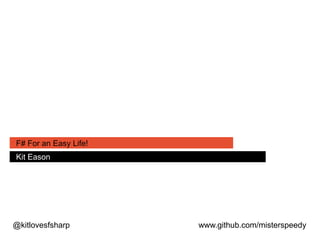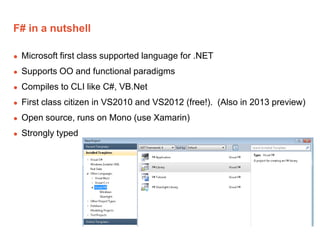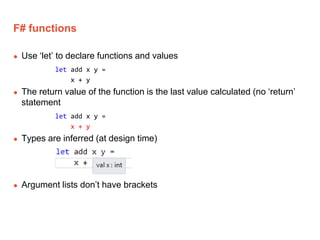Successfully reported this slideshow.

# F# Presentation for SmartDevs, Hereford

1

Share×
1 of 28
1 of 28

# F# Presentation for SmartDevs, Hereford

1

Share

An intro to F# done originally for SmartDevs, Hereford

An intro to F# done originally for SmartDevs, Hereford

## More Related Content

### Related Books

Free with a 14 day trial from Scribd

See all

### Related Audiobooks

Free with a 14 day trial from Scribd

See all

### F# Presentation for SmartDevs, Hereford

1. 1. @kitlovesfsharp www.github.com/misterspeedy F# For an Easy Life! Kit Eason
2. 2. F# in a nutshell  Microsoft first class supported language for .NET  Supports OO and functional paradigms  Compiles to CLI like C#, VB.Net  First class citizen in VS2010 and VS2012 (free!). (Also in 2013 preview)  Open source, runs on Mono (use Xamarin)  Strongly typed
3. 3. F# functions  Use ‘let’ to declare functions and values let add x y = x + y  The return value of the function is the last value calculated (no ‘return’ statement let add x y = x + y  Types are inferred (at design time)  Argument lists don’t have brackets
4. 4. F# Interactive (FSI) > let add x y = x + y;; val add : x:int -> y:int -> int > add 3 4;; val it : int = 7 > • Use F# interactive to define and try out functions
5. 5. Calculating present value > let presentValue fv r t = fv * (1.+r) ** (-t);; val presentValue : fv:float -> r:float -> t:float -> float > presentValue 2000.0 0.07 10.;; val it : float = 1016.698584 > • Formulae can be represented very directly
6. 6. The ‘map’ function  Take some collection (array, list, IEnumerable)  For every value calculate and return some other value  Result is another array/list/IEnumerable containing the results let someSquares min max = // This generates an array from min to max: let numbers = [|min..max|] Array.map (fun x -> x * x) numbers val someSquares : min:int -> max:int -> int [] > someSquares 100 110;; val it : int [] = [|10000; 10201; 10404; 10609; 10816; 11025; 11236; 11449; 11664; 11881; 12100|] >
7. 7. The forward pipe operator |>  Takes the output from the preceding function  Places it into the (last) parameter of the following function let add x y = x + y let multiply x y = x * y val add : x:int -> y:int -> int val multiply : x:int -> y:int -> int > add 2 3 |> multiply 5;; val it : int = 25 >
8. 8. The forward pipe operator |> (2)  Comes into its own when dealing with collections let rootMeanSquare min max = [|min..max|] |> Array.map (fun x -> x * x) |> Array.average |> sqrt val rootMeanSquare : min:float -> max:float -> float > rootMeanSquare -10.0 10.0;; val it : float = 6.055300708 >
9. 9. The ‘mapi’ function  Like ‘map’ but provides you with an index value 0, 1, 2 etc. let someAdditions min max = [|min..max|] |> Array.mapi (fun i x -> i + x) val someAdditions : min:int -> max:int -> int [] > someAdditions 100 110;; val it : int [] = [|100; 102; 104; 106; 108; 110; 112; 114; 116; 118; 120|] >
10. 10. Calculating present value of a cashflow  Calculate the present value of a cashflow starting at time 0 let presentValue fv r t = fv * (1.+r) ** (-t) let cfPresentValue cf r = cf |> Array.mapi (fun t amt -> presentValue amt r (float(t))) |> Array.sum val presentValue : fv:float -> r:float -> t:float -> float val cfPresentValue : cf:float [] -> r:float -> float > cfPresentValue [|1000.0; 2000.0; 2500.0|] 0.07;; val it : float = 5052.755699 >
11. 11. Great Circle Distance
12. 12. Unit Testing and TDD  Create a new F# library project  Use Nuget to bring in FSUnit  Add GreatCircleTests.fs module GreatCircleTests open NUnit.Framework open FsUnit
13. 13. Write a trivial test! module GreatCircleTests open NUnit.Framework open FsUnit [<TestFixture>] type ``Given the GreatCircleDistance function``() = [<Test>] member x.``The function returns 0 for a journey between the same points``() = let expected = 0. let actual = GreatCircle.Distance 45. 50. 45. 50. actual |> should equal expected
14. 14. Pass the test! module GreatCircle let Distance lat1 long1 lat2 long2 = 0.
15. 15. What’s the next simplest test? [<Test>] member x.``The function returns 20014 km for a journey between the poles``() = let expected = 20014. let actual = GreatCircle.Distance -90. 0. 90. 0. actual |> should equal expected
16. 16. We’re gonna need an algorithm! var R = 6371; // km var dLat = (lat2-lat1).toRad(); var dLon = (lon2-lon1).toRad(); var lat1 = lat1.toRad(); var lat2 = lat2.toRad(); var a = Math.sin(dLat/2) * Math.sin(dLat/2) + Math.sin(dLon/2) * Math.sin(dLon/2) * Math.cos(lat1) * Math.cos(lat2); var c = 2 * Math.atan2(Math.sqrt(a), Math.sqrt(1-a)); var d = R * c;
17. 17. How’s this? open System let Distance lat1 lon1 lat2 lon2 = let EarthRadius = 6378.1 let Deg2Rad deg = deg * Math.PI / 180. let lat1r = lat1 |> Deg2Rad let lat2r = lat2 |> Deg2Rad let dLat = lat2 - lat1 |> Deg2Rad let dLon = lon2 - lon1 |> Deg2Rad let a = (sin(dLat/2.) ** 2.) + (sin(dLon/2.) ** 2.) * cos(lat1r) * cos(lat2r) let c = 2. * atan2 (sqrt(a)) (sqrt(1.-a)) c * EarthRadius
18. 18. Whuuuuuuuuuuuuuuuuuu? ------ Test started: Assembly: FSUnitTestingExample.dll ------ Test 'GreatCircleTests+Given the GreatCircleDistance function.The function returns 20014 km for a journey between the poles' failed: Expected: 20014.0d But was: 20037.392103861061d at FsUnit.TopLevelOperators.should[a,a](FSharpFunc`2 f, a x, Object y) C:CodeVS2012FSUnitTestingExampleFSUnitTestingExampleGre atCircleTests.fs(28,0): at GreatCircleTests.Given the GreatCircleDistance function.The function returns 20014 km for a journey between the poles() 1 passed, 1 failed, 0 skipped, took 0.37 seconds (NUnit 2.6.1).
19. 19. They all laughed at Christopher Columbus… [<TestFixture>] type ``Given the GreatCircleDistance function``() = let margin = 0.003 [<Test>] member x.``The function returns 0 for a journey between the same points``() = let expected = 0. let actual = GreatCircle.Distance 45. 50. 45. 50. actual |> should (equalWithin margin) expected [<Test>] member x.``The function returns 20014 km for a journey between the poles``() = let expected = 20014. let actual = GreatCircle.Distance -90. 0. 90. 0. let error = expected * margin actual |> should (equalWithin error) expected
20. 20. Further tests better added as cases // Travel no distance: [<TestCase(0., 0., 0., 0., 0.)>] // Travel along the equator eastwards for 90 degrees: [<TestCase(0., 0., 0., 90., 10018.79)>] // Travel along the equator westwards for 90 degrees: [<TestCase(0., 0., 0., -90., 10018.79)>] // Travel along the equator eastwards for 180 degrees: [<TestCase(0., 0., 0., 180., 20037.58)>] // Travel along the equator westwards for 180 degrees: [<TestCase(0., 0., 0., -180., 20037.58)>] // Travel along the meridian northwards 90 degrees: [<TestCase(0., 0., 90., 0., 10018.79)>] // Travel along the meridian soutwards 90 degrees: [<TestCase(0., 0., -90., 0., 10018.79)>] // Travel from Farnham to Reigate: [<TestCase(51.214, -0.799, 51.230, -0.188, 42.6)>] // Travel from London to Sidney Australia: [<TestCase(51.51, -0.13, -33.86, 151.21, 16998.)>] member t.``the function returns the right result``(lat1, long1, lat2, long2, expected) = let actual = GreatCircle.Distance lat1 long1 lat2 long2 let error = expected * margin actual |> should (equalWithin error) expected
21. 21. Information-Rich Programming  Bring large, structured data sources into the code in a type-safe way… with Intellisense!  Implemented by ‘Type Providers’  Introduced with F#3.0 (VS2012)  Code-gen free!
22. 22. Information Rich Programming 22
23. 23. Freebase
24. 24. Nuget and Fsharp.Data
25. 25. Get airports and locations /// Gets airports between a specified start and end index (to facilitate paged access). let GetAirportsPaged startIndex pageSize = query { for airport in dc.Transportation.Aviation.Airports do where ( airport.Geolocation <> null && airport.Geolocation.Latitude.HasValue && airport.Geolocation.Longitude.HasValue ) skip startIndex take pageSize select (airport.Name, airport.Geolocation.Latitude.Value, airport.Geolocation.Longitude.Value) } |> Array.ofSeq |> Array.map (fun (name, lat, long) -> { Name = name; Lat = lat; Long = long })
26. 26. Get airports and locations (2) /// Gets all airports from Freebase which have a defined location. let GetAllAirports pageSize = let rec getPage startIndex acc = let page = GetAirportsPaged startIndex pageSize if page.Length > 0 then Array.append acc (getPage (startIndex+pageSize) page) else acc getPage 0 [||]
27. 27. Get closest airports /// Gets the closest n airports to the given airport. let GetClosest target count airportList = airportList |> Array.map (fun airport -> airport, (DistanceBetween (airport.Lat) (airport.Long) (target.Lat) (target.Long))) |> Array.sortBy (fun (airport, distance) -> distance) |> Seq.truncate count |> Array.ofSeq
28. 28. Get closest airports to a named airport /// Gets airports near an airport specified by name. let GetAirportsNear name airportList = let target = airportList |> Array.tryFind (fun airport -> airport.Name.Contains(name)) if target.IsSome then airportList |> GetClosest target.Value 20 |> Array.iter (fun (airport, distance) -> printfn "%s - %f km" airport.Name distance) else printfn "Could not find %s" name